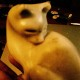# Gödel proved that there are ALWAYS more things that are true than you can prove.https://www.perrymarshall.com/articles/religion/godels-incompleteness-theorem/

Excerpt

Godel’s Incompleteness Theorem says:

“Anything you can draw a circle around cannot explain itself without referring to something outside the circle – something you have to assume but cannot prove.”

Stated in Formal Language:

Gödel’s theorem says: “Any effectively generated theory capable of expressing elementary arithmetic cannot be both consistent and complete. In particular, for any consistent, effectively generated formal theory that proves certain basic arithmetic truths, there is an arithmetical statement that is true, but not provable in the theory.”

The Church-Turing thesis says that a physical system can express elementary arithmetic just as a human can, and that the arithmetic of a Turing Machine (computer) is not provable within the system and is likewise subject to incompleteness.

Any physical system subjected to measurement is capable of expressing elementary arithmetic. (In other words, children can do math by counting their fingers, water flowing into a bucket does integration, and physical systems always give the right answer.)

Therefore the universe is capable of expressing elementary arithmetic and like both mathematics itself and a Turing machine, is incomplete.

Syllogism:

1. All non-trivial computational systems are incomplete

2. The universe is a non-trivial computational system

3. Therefore the universe is incomplete

You can draw a circle around all of the concepts in your high school geometry book. But they’re all built on Euclid’s 5 postulates which are clearly true but cannot be proven. Those 5 postulates are outside the book, outside the circle.

You can draw a circle around a bicycle but the existence of that bicycle relies on a factory that is outside that circle. The bicycle cannot explain itself.

Gödel proved that there are ALWAYS more things that are true than you can prove. Any system of logic or numbers that mathematicians ever came up with will always rest on at least a few unprovable assumptions.

Gödel’s Incompleteness Theorem applies not just to math, but to everything that is subject to the laws of logic. Incompleteness is true in math; it’s equally true in science or language or philosophy.

And: If the universe is mathematical and logical, Incompleteness also applies to the universe.

## 2 thoughts on “Gödel proved that there are ALWAYS more things that are true than you can prove.”

1.mikeoxon says:

“If the universe is mathematical and logical, Incompleteness also applies to the universe.” But – quantum theory allows events that are neither mathematical nor logical, providing they stay within boundaries defined by the uncertainty principle

Like

1.Katherine says:

I was wondering about that bit.The best bit thing is the ideaof the circle at the beginning

Like

This site uses Akismet to reduce spam. Learn how your comment data is processed.